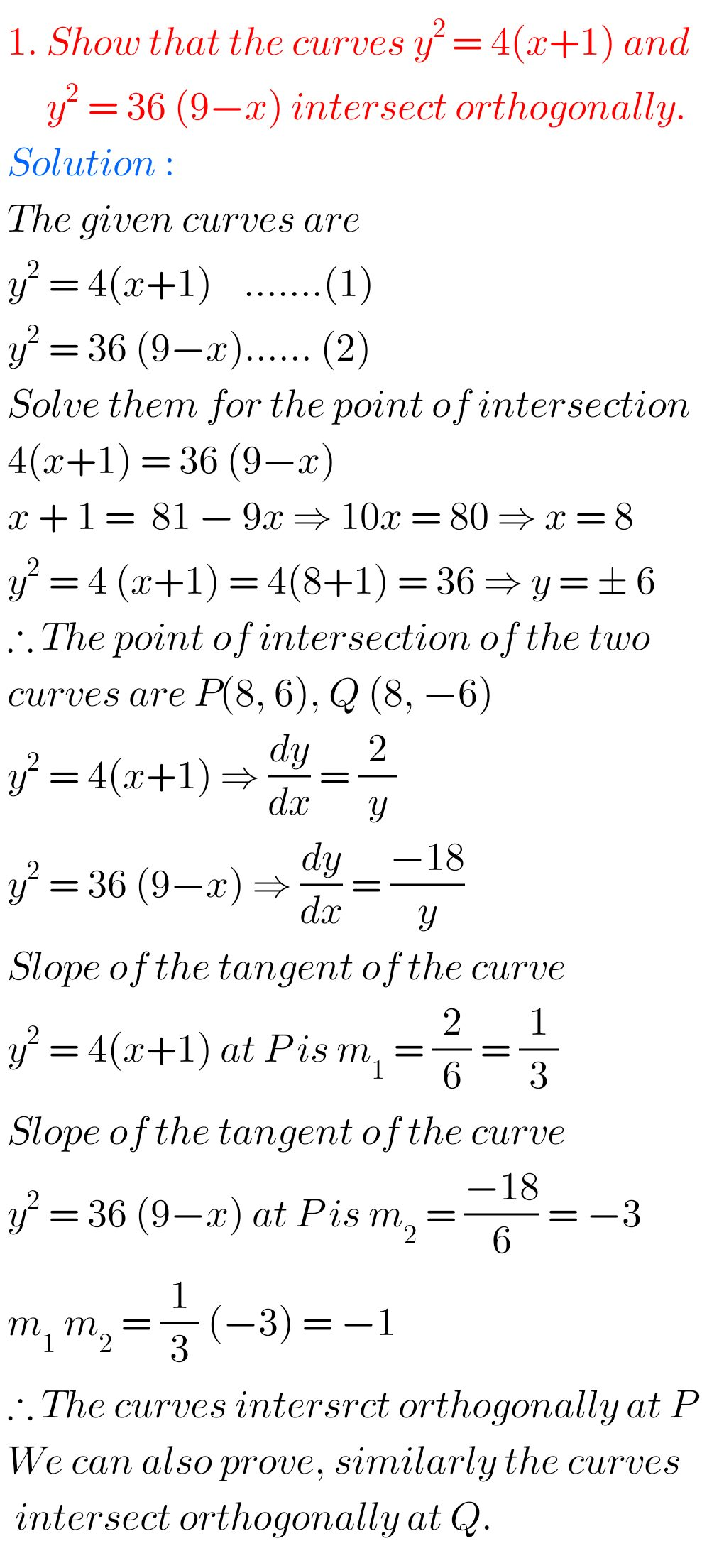# Applications of derivatives inter 1b solutions

Mathematics 1B Intermediate Applications of Derivatives solutions are given.

These solutions are very simple to understand.

Study the textbook lesson Applications of Derivatives very well.

## Intermediate mathematics first year 1b chapter 10 solutions

You can also see the solutions

SSC Maths text book Solutions class 10

Inter maths 1A textbook solutions

Inter Maths 1B textbook solutions

Inter Maths 1IA text book solutions

Inter Maths IIB text book solutions

Applications of Derivatives

Exercise 10(a)

Exercise 10(b)

Exercise 10(c)

Exercise 10(d)

Exercise 10(e)

Exercise 10(f)

Exercise 10(g)

Exercise 10(h)

You can see the solutions for some problems Applications of derivatives Intermediate first year 1b for examination purpose

10.2. Tangent and normal

10.3. Rate measure

10.4. Mean value theorems

Note : Observe the solutions and try them in your own methods.You can also see the solutions for inter 1b

1Locus

Maths real numbers solutions class 10

### 1 thought on “Applications of Derivatives Inter 1b solutions,chapter 10 Intermediate 1b solutions”

1.nice for learners